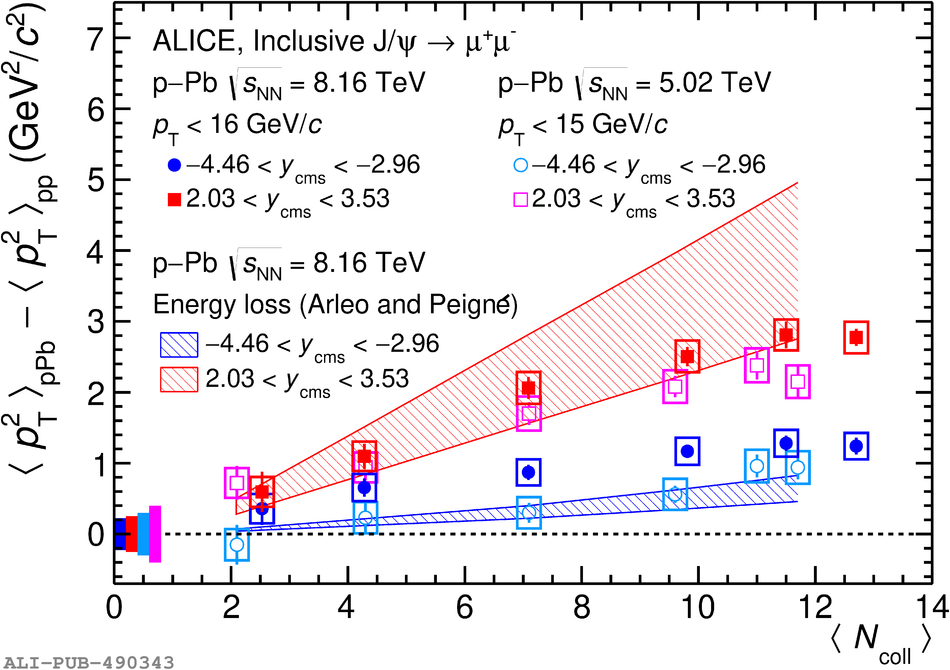# Figure 3

 \pt\ broadening of J/$\psi$, $\Delta \langle p^{2}_{\rm T}\rangle$, as a function of $\langle N_{\rm coll}\rangle$ at backward (blue circles) and forward (red squares) rapidity in p--Pb collisions at $\sqrt{s_{\rm NN}} = 8.16$ TeV compared to the results at $\sqrt{s_{\rm NN}} = 5.02$ TeV  and to energy loss model calculations . The vertical error bars represent the statistical uncertainties and the boxes around the data points the systematic uncertainties.